# Experimental Investigation on Free Surface Vortices Driven by Tangential Inlets

Experimental Investigation on Free Surface Vortices Driven by Tangential Inlets

Gianfranco CarusoLuca Cristofano Matteo Nobili Giovanni Paolo Romano

Sapienza University of Rome, Department of Astronautical, Electrical and Energy Engineering, C.so Vittorio Emanuele II, 244 – 00186 Rome, Italy

Sapienza University of Rome, Department of Mechanical and Aerospace Engineering, Via Eudossiana, 18 – 00184 Rome, Italy

Corresponding Author Email:
gianfranco.caruso@uniroma1.it
Page:
653-662
|
DOI:
https://doi.org/10.18280/ijht.340415
|
Accepted:
|
Published:
31 December 2016
| Citation

OPEN ACCESS

Abstract:

Particle Image Velocimetry (PIV) measurements have been carried out in order to analyze the structure of free surface vortices in a promoting geometry with two tangential inlets. Velocity fields associated to the free surface vortex have been obtained at different horizontal planes and Reynolds numbers. Average velocity fields have been calculated and tangential velocity profiles have been compared at different vortex stages and measurement planes. The results show that tangential flow is uniform along the vortex axis and it scales well with the average exit velocity. The tangential velocity profiles, in comparison to the potential behavior, show discrepancies especially at large distances from the vortex axis. Vorticity fields and circulation profiles have been also derived from the measured velocity fields and discussed. The circulation profiles increase along the vortex radius even at large distances from the vortex axis, so that the potential solution is not applicable at all. The comparison of tangential velocity and circulation profiles between promoted and free vortices, the last presented in a previous paper, shows that the tangential motion in a driven vortex is more intense and predominant over the sink effect (radial motion), except very close to the tank bottom, as in a forced configuration (i.e. rotating cylindrical tank).

Keywords:

Bathtub vortex, PIV, Free surface flow.

1. Introduction

A free surface vortex can be easily observed emptying a kitchen sink or industrial reservoirs. More generally, this phenomenon can affect any hydraulic intake causing important technical problems in industrial applications , . The free surface vortex formation can reduce the performance of the hydraulic devices and even damage them if floating matters or gas bubbles are entrained in the flow. The determination of the gas holdup distributions can be obtained only through complex measurement techniques . In particular, gas entrainment phenomena (GE) represent an important safety issues in Sodium-cooled Fast Reactors (SFRs), since the introduction of gas bubbles within the reactor core could result in reactivity insertion accident (RIA).

The causes and mechanisms of vortex formation are not yet completely clarified even if different researchers spent their efforts by investigating the characteristics of this physical phenomenon. Rankine  proposed a simple mathematical description for the tangential velocity, considering the flow as a forced vortex in its central core, surrounded by a free potential vortex. Burgers  provided the first exhaustive theoretical vortex model under the hypotheses of a steady, axi-symmetric, laminar, unbounded flow and negligible depth of the vortices. Other researchers (Hite and Mih , Chen et al. , Lundgren , Andersen et al. , Stepanyants and Yeoh , Suerich-Gulick et al. ) derived complex analytical vortex models and solutions starting from different simplified assumptions. However, analytical models cannot be easily applied in common industrial applications due to the usually complex boundary conditions. A different approach consists in performing numerical simulations in order to reproduce the formation and evolution of free surface vortices. Several studies (Sakai et al. , Ito et al. , Cristofano et al. ) were performed in the last years, adopting this approach, but the deformation of gas-liquid interface, combined with the swirling motion in the vortex core, makes the numerical simulation of free surface vortex extremely difficult to be performed accurately.

Therefore, in parallel, several experimental studies on free surface vortices were carried out over the years. Some of these (Gulliver and Rindels , Baum and Cook , Caruso et al. , Cristofano et al. ) were focused in individuating the critical conditions for vortex formation in terms of dimensionless parameters, so that different empirical correlations were derived. Experimental results and empirical correlations, however, strongly depend on the test specific conditions and can be hardly generalized.

Other experimental investigations were focused on performing direct measurements of the vortex velocity fields, to deeply analyze the free surface vortex structure and understand the underlying mechanisms. The Particle Image Velocimetry (PIV) technique proved to be very useful for this purpose and it was applied by many researchers in the last years .

Noguchi et al.  measured the velocity fields by means of PIV in a rotating cylindrical tank and verified that the experimental results are in good agreement with Rankine’s analytical model only when the suction rate is large. Li et al.  performed PIV measurements and compared the tangential and radial velocity distribution at different vortex stages, computing also the circulation radial profiles. Monji et al.  measured the vortex velocity fields on horizontal and vertical planes observing that, when the water level is high and the flow rate is large, the downward velocity gradient can be considered constant, as in the Burgers’ vortex model in the middle depth of the vessel. Keller et al.  performed PIV measurements in a large-scale hydraulic model, obtaining a time-averaged horizontal velocity field, the circulation profile and the gas core diameter of a free surface vortex; their results confirmed the applicability of the potential solution of the Navier-Stokes equations for a free vortex. Cristofano et al. used PIV measurements of free vortex flow fields , to validate different analytical vortex models (i.e. Burgers’, Hite’s and Chen’s) by comparing tangential and radial velocities and vorticity distributions. The free vortex is generated in a non-promoting geometry.

In , Cristofano et al. performed PIV measurements in order to characterize the structure of free vortices. Measurements were carried out at different horizontal planes and for different vortex stages, to analyze the flow field variations with the vortex strength and along the axial coordinate. It was observed that, in a free vortex, the radial flow is governed predominantly by the exit fluid velocity and it can be considered potential near the drain hole (intake). On the other hand, the tangential velocities are larger near the free surface, where also the tangential flow can be considered potential.

In the present work, the flow structure of free surface vortices, driven by tangential inlets, is analyzed by means of PIV measurements and the results are presented and discussed. A jet-driven vortex has been generated by two symmetrical tangential inlets, which provide an imposed rotation to the flow. Therefore, the analyzed vortices will be identified in the following as “forced vortices”, despite this last definition generally refers to vortices generated by a rotating tank. Measurements have been carried out in horizontal planes at different heights and for different Reynolds numbers (calculated with reference to the drain hole diameter and the mean exit velocity); varying the Reynolds number, vortices with different strength are obtained (different vortex stages). For a proper comparison between the present and the past free vortex measurements, the water level in the tank and the measurement plane heights were the same adopted in , as also the flow rates in the two vortex stage conditions. Tangential average velocity fields have been evaluated in steady-state conditions (time averaged) and compared with the potential solution (Rankine’s vortex model). Circulation profiles have been derived and analyzed. The statistical parameters of turbulence have been also calculated and presented. The specific aim of the paper is to derive a detailed comparison between the overall flow structures and the specific features of forced and free vortices, especially in terms of velocity and circulation profiles, in relation to existing vortex models.

2. Experiments

2.1 Experimental facility

Experimental tests have been carried out in the GETS (Gas Entrainment Test Section) facility; it is a closed loop with a rectangular PPMA tank, a pump, a magnetic flow meter, valves, pipes and fittings.

Fig. 1 shows the geometry of the GETS tank, which is characterized by two lateral inlets and an outlet nozzle at the center of the tank; it is 700 mm × 500 mm × 500 mm (h×l×w) with transparent walls 20 mm thick. Two vertical baffles and two other small septa are placed near the inlet zones in order to limit perturbations on the free surface due to the upward inlet flow.

In this study, a tangential inlet has been given to the fluid by placing two baffles on both sides of the tank; thus, a forced rotation, proportional to the flow rate circulating in the facility, is imposed to the flow.

## 1.png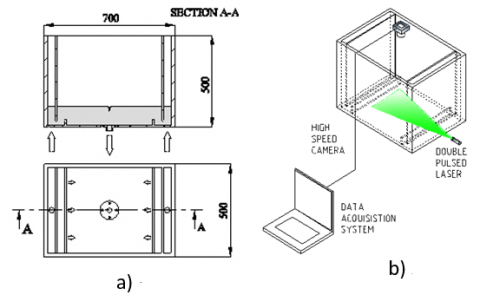Figure 1. a) Vertical section and plant of the tank, b) Schematic view of PIV apparatus

PIV measurements have been carried out at the Fluid-dynamics Laboratory of the Mechanical and Aerospace Engineering Department of the “Sapienza” University of Rome. Measurements at five horizontal planes and for three different flow rates (Table 1), corresponding to three of the four different development stages of vortex (classified as in ) have been performed. Unfortunately, it was not possible to identify a flow rate to reproduce a Stage 3 vortex in steady conditions.

The water level H in the tank was fixed at 50 mm in all the experimental tests, to compare the present results with those reported in .

For this purpose, for Stage 1 and Stage 2 conditions flow rate values are equal to those selected in ; for Stage 4, instead, a lower flow rate had to be chosen to obtain a stable vortex.

Reynolds number has been investigated between 2450 and 6360; Froude number, calculated with the exit velocity U and the water level H, is between 0.13 and 0.35. The inner diameter D of the outlet pipe is 0.026 m. The flow meter has an accuracy of 0.75% of the measured value; the measured flow rate range was 0.05-0.13 l/s.

Table 2 shows the elevation, from the tank bottom, of the horizontal measurement planes. For each plane an area of about 19 × 19 cm (about 7.3 D) was framed.

A double pulsed Nd-Yag laser, with a characteristic wavelength of the laser of 532 nm, provided a 2 mm thick light plane. The laser pulse energy was 200 mJ and the duration of the single pulse was 0.76 ms. The high-speed camera was a 10-bit CMOS BW Photron ultima APX with 1024 × 1024 pixels’ resolution at 50 fps, equipped with a Nikon Nikkor 50mm f/1.8 camera lens. Camera and laser pulses were synchronized at 50 fps for the low flow rate tests (Re≈2450) and 125 fps for the others. Therefore, the interval time between the two laser pulses and, consequently between two successive images, was respectively 1/50 s and 1/125 s.

Table 1. Development vortex stages and corresponding tested flow rates and Reynolds numbers

 Vortex stage Description Flow rate Q [l s-1] Reynolds Number Froude Number Stage 1 (S1) Surface swirl and very small dimple 0.05 ≈ 2450 ≈ 0.13 Stage 2 (S2) Well-developed dimple 0.1 ≈ 4900 ≈ 0.27 Stage 3 (S3) Bubble entraining core - - - Stage 4 (S4) Full air core 0.13 ≈ 6360 ≈ 0.35

Table 2. Horizontal measurement planes

 Laser plane ID Plane elevation z [mm] Dimensionless elevation z*=z/H Plane 1 (P1) 15 0.3 Plane 2 (P2) 25 0.5 Plane 3 (P3) 35 0.7 Plane 4 (P4) 45 0.9

Two single images resulting from each double pulse have been cross-correlated to obtain a single frame. The laser repetition rate of a double pulse, which coincides with the time resolution of the system, was 5 Hz. The total acquisition time for each test was 204 s (the maximum time allowable by the internal memory of the camera), corresponding to about 1000 images.

The internal tank surfaces have been obscured with a black adhesive film, leaving the tank interior optically accessible only through a window on the frontal wall for laser entrance. As shown in Fig. 1, the camera was placed above the tank and the whole experimental apparatus was covered with a black sheet, to reduce the reflections and enhance the image quality. Hollow glass spheres with a diameter of 10 µm were used as seeding.

Each test condition has been identified as “SXPY”, where X and Y are, respectively, the stage (S) number and the measuring plane (P) number.

Experimental tests have been carried out following a specific procedure already adopted in , because of the strong influence of initial conditions on free surface vortex dynamics.

After a previous test or perturbation, a waiting time (not less than 10 minutes) has been foreseen before turning on the pump; then, before starting PIV acquisitions, additional five minutes have been waited to ensure that all disturbances due to the initial transient vanished.

2.2 Data post-processing

Strong undesired reflections affected the acquired images; therefore, a background subtraction has been applied to reduce the background noise. The PIV analysis has been performed in two iterations: the first one with a starting window size equal to 128 x 128 pixels with 75% overlapping, the second one with a starting window size 32 x 32 pixel and 50% overlapping. The vector spacing was 16 pixels, corresponding to about 3 mm (0.115 D).

Unlike the experimental tests reported in , it was not possible to identify the vortex center of each instantaneous velocity field because of the “lens effect” caused by the free surface deformation. The tangential inlets, in fact, generate a much more intense vortex and the deformation of the free surface affects a larger zone around the vortex axis, introducing measurement errors near the vortex center even in the measurement planes not crossing vortex air core (the camera is placed above the tank, thus the free surface is always between the camera and the measurement plane, as can be seen from Fig. 1b.

However, in the present study, the tangential inlets stabilize and keep the occurred vortices centered with respect to the intake pipe. Therefore, an Eulerian average operation has been performed on the 1024 frame of each test case to calculate the average velocity fields. Consequently, in this study the average velocity field dimension is 63×63, as for all the instantaneous frames.

Average velocity fields and profiles, vorticity distributions, circulation profiles and Root Mean Square (RMS) of tangential velocity profiles were calculated assuming a cylindrical coordinate system referred to the actual center of the vortex.

3. Experimental Velocity Fields in Forced Vortices

3.1 Average velocity fields

The processed images have been used for each test to derive time-averaged velocity components $\overline{u}(x, y)$ and $\overline{v}(x, y)$ in x- and in y- directions.

Average errors in measurements have been determined by the statistical error $\sigma_{u_{j}}^{s}$ on the velocity component $u_{j}$, which is proportional to the standard deviation $\sigma_{u_{j}}$ :

$\sigma_{u_{j}}^{s}(x, y)=\frac{2 \cdot \sigma_{u_{j}}}{\sqrt{N}}$(1)

In most experimental measurements, the laser sheet cut the vortex gas core, generating strong reflections worsening image quality and measurement accuracy. Near the free surface, reflections are even more relevant because the vortex air core is larger than in free vortex conditions. Moreover, when the laser sheet is reflected by the vortex air core, the zone behind the vortex is no longer lighted. Therefore, this “vortex shadow” does not allow to perform measurement in that zone because seeding particles cannot be detected.

In Fig. 2, the average velocity vector fields for two vortex stages (S1 and S4) and for two horizontal planes (P1 and P3) are reported; the contours of the vorticity in z- direction, normalized by the average exit velocity U and the drain hole diameter D, are showed in background color.

The vector length is proportional to the velocity magnitude on the measurement plane. The coordinates x* and y* are normalized with respect to the radius of the intake (D/2). The direction of the two tangential inlets is parallel to the x - axis and the generated rotation is counterclockwise. From Fig. 2 it can be clearly seen that the center of rotation of the vortex is never detectable because of the lens effect caused by the deformed interface. The direction of the laser light is parallel to the y- axis and it comes from y* < 0 in the figures. Accordingly, the area where y* <0 is well lit, while for the measurement planes closer to the free surface (P3 and P4) the “vortex shadow” and the reflections introduced by the deformed free surface do not allow a fully reliable measure of the velocity field for y* > 0. More intense vortices (S4, Fig. 2b and Fig. 2d) cause more inaccuracies. In most cases, it was possible to identify the boundary of the vorticity accumulation zone (or at least part of it). The tangential inlets impose a rotational motion that extends throughout the measurement area even for the less intense vortex stage (S1). However, larger Reynolds numbers (plots in the second column of the figure) and, therefore, more intense vortices, result in higher velocities of the fluid, as can be noted from the vector length in Fig. 2. Moreover, the radius of vorticity accumulation zone is almost the same at different measurement planes, while it increases for more intense vortices (thus for increasing Reynolds number). Therefore, the vortex size is constant along the axial coordinate and increases with Reynolds number.

## 2.pngFigure 2. PIV average velocity fields in vector form with normalized vorticity magnitude contour for cases: a) S1P4; b) S4P4, c) S1P2, d) S4P2

The average velocity fields for the cases related to the highest measurement plane P4 are shown in Fig. 3; two circumferences are highlighted (the inner one with a radius equal to the radius of the drain hole R = 0.013 m, the outer one with the radius equal to twice that radius). Referring to these two circumferences, it is possible to evaluate the width of the area affected by optical errors, where a reliable measurement of the velocity field cannot be obtained. In cases S1P4 and S2P4 errors extend approximately up to r*=1 (r* is the normalized distance with respect to the radius of the drain hole r* = r/R), while for the case S4P4 errors extend also beyond the red circle, especially where y* ≈ 0. Therefore, velocity fields and experimental profiles can be reasonably considered reliable for r*>1 for cases S1 and S2, and for r* > 2 for cases S4 (at least for the half-plane where y* < 0).

## 3.png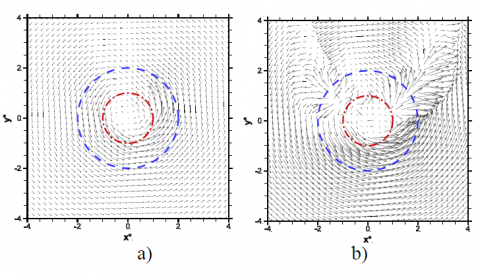Figure 3. Average velocity fields at P4 for cases a) S1 and b) S2. The inner and the outer circles have a radius of D/2 and D, respectively

3.2 Average velocity profiles

To extract the radial and tangential velocity profiles, the velocity fields were transformed from the Cartesian coordinate system$(\overline{u}, \overline{v})$ to a polar coordinate system $\left(\overline{u_{r}}, \overline{u_{\theta}}\right)$ and the average operation has been performed on different angular positions:

\begin{aligned}\left\langle\overline{u_{\theta}}(r)\right\rangle &=\frac{1}{N} \sum_{i=1}^{N} \overline{u_{\theta_{i}}}(r, \theta) \\\left\langle\overline{u_{r}}(r)\right\rangle &=\frac{1}{N} \sum_{i=1}^{N} \overline{u_{r_{i}}}(r, \theta) \end{aligned}(2)

Due to vortex effects on the propagation of light and considering the symmetry of the flow field on the plane, the averaged velocity profiles are limited to the half-plane y* <0, so that they are evaluated over 180°. In any case, to verify the effectiveness of this assumption, two test cases with negligible optical deformation effects (S1P1 and S4P2) were considered and velocity profiles obtained after an azimuthal average over 180° and 360° were compared. Fig. 4 shows this comparison for the tangential velocity component, normalized with respect to the average velocity at the intake U. The shaded areas limit the zone of possible optical effects, where also the velocity profiles are dashed without error bars to indicate that low measurement accuracy. From the figure, it can be noted that the two average profiles are very close to one another, leading to considering appropriate for tangential velocity profiles the azimuthal averaging over 180° rather than 360°.

Radial velocity profiles obtained averaging on 180°, instead, show important differences compared with those obtained from the 360° average in the same conditions (not shown here). This occurs probably because the radial motion is much less intense than the tangential one, thus even small errors can alter the average profile. Considering this fact and the relative secondary importance of this component in forced vortices, results concerning radial velocities are not reported here.

In Fig. 5, the experimental profiles of tangential velocity are shown separately for the three vortex stages at different measuring plane heights (P4 near the free surface, P1 near the bottom of the tank), normalized with respect to the average velocity U at the intake. The radial coordinate is normalized with the intake pipe radius R (r* = r/R).

## 4.pngFigure 4. Comparison between tangential velocity profiles averaged on 180° and 360° for cases: a) S1P1, b) S4P2

The shaded areas in figures demarcate the zone where optical errors could not allow reliable measurements. The tangential velocity profiles show the typical trend with a linear inner region (in the shaded zone) and hyperbolic decay in the outer zone, as in the Rankine’s vortex model.

## 5.png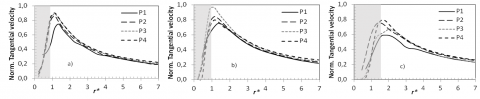Figure 5. Normalized tangential velocity profiles at different measurement planes for cases: a) S1, b) S2, c) S4

A residual rotation even at large r* values is always present, provided by the tangential inlets. It can be noted that, for each vortex stage, the normalized tangential velocity profiles measured at different planes are very close one to another, thus suggesting that the tangential flow is uniform along the axial coordinate and that it is not influenced by the effects of the sink even at a distance of about 0.015 m (≈0.5D) from the tank bottom (the height of the lower plane P1). For all the cases S1 and S2, the maximum tangential velocity is reached at r* ≈ 1, thus, the size of the linear inner zone is almost equal to the intake radius and it is almost the same at the different heights. Moreover, the normalized tangential velocity peak assumes a value close to 1, for the cases S1 and S2, so that the maximum tangential velocity scales quite well with the average velocity at the drain hole. For the full air core (case S4), the peak in tangential velocity is in the shaded zone, where data cannot be considered fully reliable. The average statistical error of tangential velocities is about 1%, with a maximum of about 6% (out of the shaded zones).

In Fig. 6, the tangential velocity profiles in the measurement planes P4 and P2 for three different vortex stages are presented. The darker shaded zone is for S1 and S2 cases while the lighter refers to S4 cases. It is evident that the normalized profiles, which are related to different flow rates, are quite close, confirming the good scaling behavior with respect to the average exit velocity U. For all measurement planes, however, the tangential velocity profiles, related to the higher Reynolds number (S4), assume larger values in the region where measurements are reliable (r* > 2). As expected, the residual rotation at large r* increases with Reynolds number (thus, with the flow rate).

## 6.pngFigure 6. Normalized tangential velocity profiles for the three vortex stages at planes: a) P2, b) P4

A comparison between the measured tangential velocity profiles and the potential behavior predicted by the Rankine vortex model  has been performed. The expression proposed by Rankine for the tangential velocity is:

$u_{\theta}=\frac{\Gamma_{\infty}}{2 \pi r}$(3)

where $\Gamma_{\infty}$ is the overall vortex circulation. The configuration of the tank with the tangential inlets provide an increasing behavior of the circulation profile with the radial coordinate (which will be shown in next section and in Fig. 8). The input circulation $\Gamma_{\infty}$  in Eq (3) has been computed for each test case according to a method specified in  and used by Sakai et al. . Following this method, the isoline of the second invariant of the velocity deformation tensor is determined. The second invariant corresponds to a negative value of the pressure Laplacian and it changes the value from plus to minus near the vortex center. In the evaluation, the zero value of the second invariant is searched near the liquid surface the value $\Gamma_{\infty}$ is evaluated as that circulation computed at a radial distance equal to twice the radius of the zero-isoline (see next section, Eq. (4)). Circulation values evaluated for the different tests are reported in Table 3.

Table 3. $\Gamma_{\infty}$ values for the different test cases

 Test Case [m2s-1]  x102 Test Case [m2s-1] x102 Test Case [m2s-1] x102 S1P4 1 S2P4 2.27 S4P4 3.31 S1P3 1 S2P3 2.26 S4P3 3.31 S1P2 0.96 S2P2 2.15 S4P2 3.23 S1P1 0.96 S2P1 2.21 S4P1 2.98

## 7.pngFigure 7. Tangential velocity profiles compared with Rankine model for cases: a) S1, b) S2, c) S4

In Fig. 7 all the experimental tangential velocity profiles (normalized) are compared with the potential behavior derived as explained before, using the average circulation value in each vortex stage. It can be noted that there is a quite good agreement between measured and predicted tangential velocity profiles even though, in all cases, in the outer zone (about r* > 4) potential profiles are always below the corresponding experimental ones. This is in accordance with the fact that the circulation profiles increase with the radial distance, without reaching a constant value.

4. Circulation

As described in the previous section, from the average velocity fields it is possible to calculate the circulation on a closed path, C, around the vortex. The circulation is a measure of vortex strength and it is related to the vorticity component orthogonal to the plane $\omega_{z}$ through the Stokes’ theorem:

$\Gamma=\oint_{C} \vec{u} \cdot d \vec{l}=\int_{S} \omega_{z} d S$(4)

where S is the surface in the horizontal plane enclosed by the line C and $d \vec{l}$ is an elementary path on C. In Fig. 8 and Fig. 9 the circulation profiles, normalized by the circulation number ($N_{\mathrm{r}}=\Gamma D / Q$ ) are shown, respectively, for different measurement planes and different vortex stages. The radial distance is normalized by the drain hole radius (r*= r/R). The circulation profiles are characterized by a rapid increase in the zone around the vortex axis (r* < 1), an evident elbow, and another almost linear increasing behavior with the radial coordinate in the outer zone, with a lower slope. The highest values of normalized circulation are measured near the free surface, even if the circulation profiles related to the different measurement planes are fairly close. On the same measurement plane, the circulation increases with increasing flow rate.

## 8.png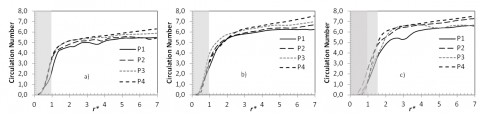Figure 8. Normalized circulation profiles at different measurement planes for cases: a) S1, b) S2, c) S4

## 9.png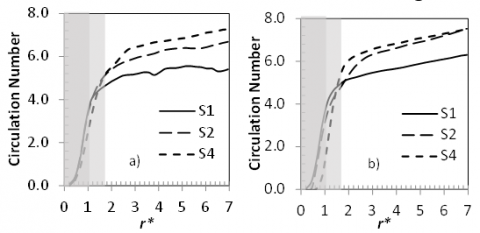Figure 9. Normalized circulation profiles for different vortex stages at planes: a) P1, b) P4

Compared with the potential behavior, which reaches a constant circulation value in the outer zone, the experimental circulation profiles continue to increase radially in the outer zone (large r*), this trend being caused by the tangential inlets.

The values of circulation, computed for each case according to the method proposed by Sakai et al. in , employed in previous section to derive the potential profiles of tangential velocity, have been also used here to show the circulation number $N_{\Gamma}=\Gamma D / Q$ as a function of Reynolds number, Re, and of the height of the measuring plane z* (normalized by the level of water in the tank, H = 50 mm). As reported in Fig. 10, it can be noted that the circulation of the vortex increases with Reynolds number (and thus mainly with the flow rate) at constant measurement plane height. On the other hand, at constant Reynolds number the circulation slightly increases with the measurement plane height, thus confirming that the tangential motion has the same intensity all over the tank, at least for z* > 0.3.

## 10.png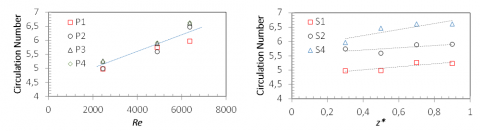Figure 10. Circulation number Vs: a) Reynolds number, b) Normalized height of the measurement plane

5. Forced and Free Vortex Velocity Fields Comparison

In this section, the flow field of the forced vortices are compared with free vortices, whose results were presented in , the comparison being made in terms of tangential velocities and circulation for vortex stages S1, S2 and S4.

The flow rates Q related to S1 and S2 cases are the same in the forced and free vortices tests (see Table 4), whereas stability problems occurred in stage S4 led to choose a lower flow rate in the present tests, compared to the free vortex cases, as reported in Table 4. The measurement planes P4, P3 and P2 are the same, while the position P1 is available only for the present forced vortex tests.

Table 4. Flow rates and Reynolds numbers of forced and free vortex test conditions

 Vortex stage Flow rate Q [l s-1] Reynolds Number Stage 1 (S1) 0.05 ≈ 2450 Stage 2 (S2) 0.1 ≈ 4900 Stage 4 (S4) 0.13 (forced) 0.23 (free) ≈ 6360 (forced) ≈ 11260 (free)

## 11.png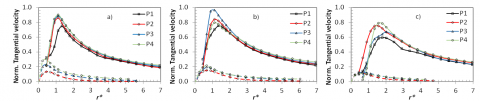Figure 11. Comparison between the normalized tangential velocity profiles of forced (solid lines) and free (dashed lines) vortices at the measurement planes: a) S1, b) S2, c) S4

Fig. 11 shows the tangential velocity profiles for forced (solid lines) and free (dashed lines) vortices, at the different vortex stages and measurement plane heights. Velocities and radial coordinates are normalized, respectively, by the average exit velocity of the fluid U and the intake radius R. As expected, for all vortex stages the tangential flow is much more intense in forced vortices, since the tangential inlets impose an additional rotational motion to the fluid. Normalized tangential velocity peaks reaches values of about 1 in forced vortices, indicating that average exit velocity can be a quite good scaling parameter for tangential flow for that kind of vortices. The imposed rotation keeps uniform the tangential flow along the axial coordinate, being predominant over the sink effect even at short distances from the tank bottom (P1). On the other hand, in free vortices, the presence of the intake on the bottom affects the flow even at the highest measurement plane (plane P3, i.e. at 0.035 m from the intake). It is also evident that, in forced vortices, the tangential flow involves a larger zone around the vortex axis (with a wider linear inner zone) and it does not vanish at large r* values.

Therefore, in this condition the boundary effects are much more important and must be considered both in experiments and numerical simulations.

The profiles of the circulation number of forced (solid lines) and free (dashed lines) vortices are compared in Fig. 12, the circulation being normalized by the average velocity U in the outlet and the diameter of the drain hole D. As it can be seen, the tangential inlets result in larger circulation values for all vortex stages compared to free vortices. Moreover, as already noticed, the circulation profiles are slightly increasing with the radial coordinate at the different heights for forced vortices, while the circulation profiles reach a constant (near the free surface) or a decreasing behavior in free vortices, thus confirming a larger dependence of the boundary conditions in comparison to the free case.

From the circulation profiles, the dimension of the vortex can be estimated by comparing the radial coordinate of the profile elbow. It can be seen that, at a fixed flow rate and height, the dimension of forced vortices is about twice larger (as expected) than the free vortex one.

## 12.pngFigure 12. Comparison between the normalized circulation profiles of forced (solid lines) and free (dashed lines) vortices at the measurement planes for: a) S1, b) S2

6. Turbulence Intensity

The Root Mean Square (RMS) values of the tangential velocity component fluctuations have been calculated to evaluate the turbulence intensity:

$u_{\theta}^{\prime}=\sqrt{\frac{\sum_{i=1}^{N}\left(u_{\theta_{i}}(x, y, t)-\overline{u_{\theta}}(x, y)\right)^{2}}{N}}$(5)

RMS values have been normalized by the exit velocity U, and obtained by applying the same average operation used for velocity profiles (average on 180°).

In Fig. 13, the profiles of the normalized RMS of the tangential velocity are shown for all vortex stages at the different measurement planes. As can be seen from the figure, an increasing behavior of the RMS tangential velocity profiles can be observed in the inner zone (r* < 2) for cases S1 and S2, due to the presence of the tangential velocity gradients in this zone (see Fig. 5). The same trend can be observed for S4 cases in the shaded area. For r* > 2, the more intense is the vortex (i.e. high Reynolds numbers), the larger are the RMS values.

## 13.png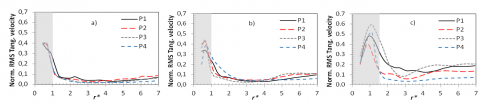Figure 13. Profiles of normalized tangential velocity RMS at the measurement planes for: a) S1, b) S2, c) S4

The RMS profiles show also a constant or slightly increasing trend with the radial coordinate. The normalized RMS profiles are quite independent on the measurement plane height for S1 cases, while for S2 and S4 there is a higher dependence. The larger values of RMS are those related to planes P3 and P2 (Fig. 13b and 13c), while the RMS profiles related to plane P4, where the tangential velocity is higher, are characterized by lower fluctuations. This behavior could be explained by considering that the height of the tangential inlet lower boundary is halfway between planes P3 and P2. Therefore, in this mixing zone, between the flow coming from the tangential inlets and the water in the tank, the shear stresses cause strong fluctuations, with a consequent increasing of the turbulence intensity. On the other hand, at P4 the flow provided by the tangential inlets is more stable and the fluctuations of tangential velocity are small. In the region where r* > 2, the intensity of the fluctuations does not exceed 10% for cases S1 and S2, while fluctuation intensity reaches values equal to about 20% for cases S4. RMS profiles show some peaks near the vortex axis, probably caused by optical effects due to the free surface deformation.

7. Conclusions

The flow fields of forced free surface vortices have been investigated through PIV measurements, performed at four horizontal planes and for different vortex stages. The forced vortices were generated in a promoting tank geometry, by imposing a rotation to the fluid through two tangential inlets. The observed vortices were steady and centered at the position of the drain hole throughout the tests, indicating that the tangential inlets stabilized the occurred vortices compared to free vortices. From the measured velocity fields, it turned out that the radial flow is poorly developed at all the analyzed heights and is highly influenced by optical errors due to free surface deformation. The tangential flow, instead, is uniform along the axial coordinate and it is not influenced by the effects of the sink, even at about 0.015 m from the tank bottom. The normalized tangential velocity peaks assumed a value close to 1, for the cases S1 and S2, indicating that the average exit velocity, which has been used to normalize the velocity profiles, could be a good scaling parameter for tangential flow in a forced vortex. The measured tangential velocity profiles, compared with the potential profiles predicted by Rankine’s vortex model, showed that tangential flow can be considered potential in the first part of the inverse radius decay of the tangential velocity (about r* < 4), but, in the outer zone, potential profiles are always below the corresponding experimental ones. This discrepancy is related to the behavior of the circulation profiles. In fact, the circulation profiles show a linear increasing behavior at large r* values, while a potential vortex is characterized by a constant circulation value in the outer zone. The circulation of the analyzed forced vortices increases with the Reynolds number at constant measurement plane height; it also increases, slightly, with the measurement plane height at a constant Reynolds number.

The forced vortex flow fields, measured with PIV techniques, were compared with those related to free vortices, generated in the same test facility but without tangential inlets. The water level in the tank and the exit pipe diameter were the same for all test cases (of forced and free vortices), for cases S1 and S2 also the imposed flow rates were the same. As expected, for all the vortex stages, the tangential flow is much more intense in forced vortices, since the tangential inlets impose a rotational motion to the fluid. Moreover, the tangential flow involves a larger zone around the vortex axis and it does not vanish at large r* values, due to a strong boundary effect. Normalized tangential velocity peaks reaches higher values (about 1) in forced vortices. The imposed rotation seemed to be predominant over the sink effect even at short distances from the tank bottom (P1), while, in free vortices, the presence of the intake on the bottom starts influencing the flow even far from the outlet (plane P3 at 0.035 m). Concerning the comparison of the circulation profiles, the tangential inlets resulted in larger circulation values for all vortex stages compared to free vortices. Moreover, the circulation profiles were slightly increasing with the radial coordinate at the different heights for forced vortices, while the circulation profiles reached a constant (near the free surface) or decreasing behavior in free vortices. The dimension of the vortex, considered as the radial coordinate of the profile elbow, is much larger for forced vortices, as expected, at a fixed flow rate and height, compared to free vortices.

Nomenclature
 D, R drain hole diameter, radius, m H water level, m $N_{\Gamma}$ circulation number $=\Gamma D / Q$, - Q flow rate, m3 s-1 r distance from the drain hole center, m r* adimensional radius = r/R, - u, U velocity, exit velocity, m s-1 x, y, z cartesian coordinates, m x*, y*, z* adimensional coordinates, - Greek symbols $\Gamma$ circulation, m2. s-1 Ɵ angular coordinate $\sigma$ standard deviation $\omega$ vorticity, s-1 Subscripts Ɵ tangenzial r radial z axial
References

 X. Zhang, “Hydraulic characteristics of rotational flow shaft spillway for high dams,” Int. J. of Heat and Technology, vol. 33, no. 1, pp. 167-174, 2015. DOI: 10.18280/ijht.330123.

 Sivakumar, K., Natarajan, E. and Kulasekharan, N. “Experimental studies on turbulent flow in ribbed rectangular convergent ducts with different rib sizes,” Int. J. of Heat and Technology, vol. 32, no. 1-2, pp. 79-85, 2014.

 T. Schafer, A. Bieberle, M. Neumann and U. Hampel, “Application of gamma-ray computed tomography for the analysis of gas holdup distributions in centrifugal pumps,” Flow Meas Instrum, vol. 46, pp. 262-267, 2015. DOI: 10.1016/j.flowmeasinst.2015.06.001.

 W.J.M. Rankine, Manual of Applied Mechanics, Griffin, London, 1858.

 J.M. Burgers, “A mathematical model illustrating the theory of turbulence,” Adv In Appl Mech, pp. 171-199 New York: Academic Press, 1948.

 E.J. Hite and W.C. Mih, “Velocity of vertical vortices at hydraulic intakes,” J Hydr Eng-ASCE, vol. 120, no. 3, pp. 284-297, 1994. DOI: 10.1061/(ASCE)0733-9429(1994)120:3(284).

 Y.I. Chen, C. Wu, M. Ye and X. Ju, “Hydraulic characteristics of vertical vortex at hydraulic intakes,” J Hydrodyn, vol. 19, no. 2, pp. 143–149, 2007. DOI: 10.1016/S1001-6058(07)60040-7.

 T. S. Lundgren, “The vortical flow above the drain-hole in a rotating vessel,” J Fluid Mech, vol. 155, pp. 381–412, 1985. DOI: 10.1017/S0022112085001860.

 A. Andersen, T. Bohr, B. Stenum, J. Jull Rasmussen and B. Launtrup, “The bathtub vortex in a rotating container,” J Fluid Mech, vol. 556, pp. 121-146, 2006. DOI: 10.1017/S0022112006009463.

 Y.A. Stepanyants and G.H. Yeoh, “Stationary bathtub vortices and a critical regime of liquid discharge,” J Fluid Mech, vol. 604, pp. 77-98, 2008.

 F.J. Suerich-Gulick, S. Gaskin, M. Villeneuve and E. Parkinson, “Free surface intake vortices: scale effects due to surface tension and viscosity,” J Hydraul Res, vol. 52, no. 4, pp. 513-522, 2014. DOI: 10.1080/00221686.2014.905503.

 T. Sakai, Y. Eguchi, H. Monji, K. Ito and H. Ohshima, “Proposal of design criteria for gas entrainment from vortex dimples based on a computational fluid dynamics method,” Heat Transfer Eng, vol. 29, pp. 731-739, 2008. DOI: 10.1080/01457630801981739.

 K. Ito, T. Kunugi, H. Ohshima and T. Kawamura “Formulations and validations of a high-precision volume-of-fluid algorithm on nonorthogonal meshes for numerical simulations of Gas Entrainment phenomena,” J Nucl Sci Technol, vol. 46, pp. 366-373, 2009. DOI: 10.1080/18811248.2007.9711542.

 L. Cristofano, M. Nobili and G. Caruso, “Numerical evaluation of gas core length in free surface vortices,” J Phys: Conf Ser, vol. 547, art. n. 012030, 2014. DOI: 10.1088/1742-6596/547/1/012030.

 J.S. Gulliver and A.J. Rindels, “Weak vortices at vertical intakes,” J Hydr Eng-ASCE, vol. 113, no. 9, pp. 1101-1116, 1987. DOI: 10.1061/(ASCE)0733-9429(1987)113:9(1101).

 M.R. Baum and M.E. Cook, “Gas entrainment at the free surface of a liquid: entrainment inception at a laminar vortex,” J Brit Nucl Eng Soc, vol. 13, pp. 203-209, 1974.

 G. Caruso, L. Cristofano, M. Nobili and D. Vitale Di Maio, “Experimental investigation of free surface vortices and definition of Gas Entrainment occurrence maps,” J Phys Conf Ser, vol. 501, art. n. 012019, 2014. DOI: 10.1088/1742-6596/501/1/012019.

 L. Cristofano, M. Nobili and G. Caruso, “Experimental study on unstable free surface vortices and gas entrainment onset conditions,” Exp Therm Fluid Sci vol. 52, pp. 221-229, 2014. DOI: 10.1016/j.expthermflusci.2013.09.015.

 J. Keller, G. Moller and R.M. Boes, “PIV measurements of air-core intake vortices”. Flow Meas Instrum, vol. 40, pp. 74-81, 2014. DOI: 10.1016/j.flowmeasinst.2014.08.004.

 T. Noguchi, S. Yukimoto, R. Kimura and H. Niino, “Structure and instability of a sink vortex,” in Proc. PSFVIP-4, Chamonix, France, 2003.

 H. Li, H. Chen, Z. Ma and Y. Zhou, “Experimental and numerical investigation of free surface vortex,” J Hydrodyn Ser B, vol. 20, no. 4, pp. 485-491, 2008. DOI: 10.1016/S1001-6058(08)60084-0.

 H. Monji, T. Shinozaki, H. Kamide and T. Sakai, “Effect of experimental conditions on gas core length and downward velocity of free surface vortex in cylindrical vessel,” J Eng Gas Turb Power, vol. 132, no. 1, art. n. 012901, 2010. DOI: 10.1115/1.3078704.

 L. Cristofano and M. Nobili, “Validation of free surface vortex analytical models,” in Proc. 5th Int. Youth Conf. on Energy – IYCE 2015, art. n. 7180741, 2015. DOI: 10.1109/IYCE.2015.7180741.

 L. Cristofano, M. Nobili, G.P. Romano and G. Caruso, “Investigation on bathtub vortex flow field by Particle Image Velocimetry,” Exp Therm Fluid Sci, vol. 74, pp. 130-142, 2016. DOI: 10.1016/j.expthermflusci.2015.12.005.

 J.C.R. Hunt, A.A. Wray and P. Moin, “Eddies, stream, and convergence zones in turbulent flows,” Center for Turbulence Research Report CTR-S88: 193–208, 1988.